HISTOGRAM METHOD - Web application - Optimization method - In a nutshell - Original papers - Presentation - Interactive explanation - FAQ - How it works - Program codes Links - Histogram Optimization - Kernel Optimization - State-space analysis - Point process - HOME Page
 Histogram Bin-width Optimization Web application for optimizing a histogram

 Shimazaki and Shinomoto. Neural Comput, 2007, 19(6), 1503-1527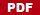## Web Application for Bin-width Optimization (Ver. 2.0)

 1. Copy&Paste your data 4.37 3.87 4.00 4.03 3.50 4.08 2.25 4.70 1.73 4.93 1.73 4.62 3.43 4.25 1.68 3.92 3.68 3.10 4.03 1.77 4.08 1.75 3.20 1.85 4.62 1.97 4.50 3.92 4.35 2.33 3.83 1.88 4.60 1.80 4.73 1.77 4.57 1.85 3.52 4.00 3.70 3.72 4.25 3.58 3.80 3.77 3.75 2.50 4.50 4.10 3.70 3.80 3.43 4.00 2.27 4.40 4.05 4.25 3.33 2.00 4.33 2.93 4.58 1.90 3.58 3.73 3.73 1.82 4.63 3.50 4.00 3.67 1.67 4.60 1.67 4.00 1.80 4.42 1.90 4.63 2.93 3.50 1.97 4.28 1.83 4.13 1.83 4.65 4.20 3.93 4.33 1.83 4.53 2.03 4.18 4.43 4.07 4.13 3.95 4.10 2.72 4.58 1.90 4.50 1.95 4.83 4.12 2. This may take some time. 3. 4. *The data is processed on your own computer (the data is NOT transferred to our server). *The program searches number of bins from 2 to 200. 07/06/16 Ver. 1.0 06/11/08 Ver. 0.10 Improved data sheet output. Web Application for Histogram Bin-width Optimization © 2006-2011 Hideaki Shimazaki

## Histogram Binwidth Optimization Method

 Shimazaki and Shinomoto, Neural Comput 19 1503-1527, 2007 I. Divide the data range intobins of width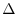. Count the number of events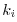that enter the i'th bin. II. Calculate the mean and variance of the number of events as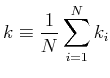and* III. Compute a formula*,IV. Repeat i-iii while changing. Find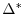that minimizes. *VERY IMPORTANT: Do NOT use a variance that uses N-1 to divide the sum of squared errors. Use the biased variance in the method. *To obtain a smooth cost function, it is recommended to use an average of cost functions calculated from multiple initial partitioning positions.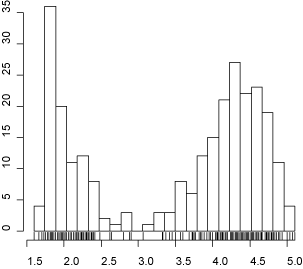An Optimized Histogram. Data: the duration for eruptions of the Old Faithful geyser in Yellowstone National Park (in minutes)

## Histogram Optimization in a NutshellAn unknown underlying rate that generates observable events. Event sequences generated from the unknown rate. Histograms constructed from the events. It is not obvious which bin size should be used. Mean Integrated Squared Error (MISE), a measure of the goodness-of-the-fit of a histogram to the unknown rate. A histogram with the bin size that minimizes the MISE is optimal. Note that we can not directly compute the MISE since we do not know the underlying rate. However, the MISE can be estimated from the data. The optimal bin size can be estimated as the one that minimizes the estimated MISE.

### Original papers

 From the observed data only, the method estimates a binwidth that minimizes expected L2 loss between the histogram and an unknown underlying density function. An assumption made here is merely that samples are drawn from the density independently each other. The method was developed in collaboration with Prof. Shinomoto in Kyoto University.Download full paper Shimazaki H. and Shinomoto S., A method for selecting the bin size of a time histogram Neural Computation (2007) Vol. 19(6), 1503-1527Short paper Shimazaki H. and Shinomoto S., A recipe for optimizing a time-histogram Neural Information Processing Systems (2007) Vol. 19, 1289-1296Presentation Slides and FAQNew original paper on Kernel Density Estimation Shimazaki H. and Shinomoto S., Kernel Bandwidth Optimization in Spike Rate Estimation. Journal of Computational Neuroscience (2009) doi:10.1007/s10827-009-0180-4 [Open Access: PDF, LINK] Go to Kernel Bandwidth Optimization page.

 pdf

## What is an Optimal Bin Size?

 When you make a histogram, you need to choose a bin size. How large (or small) the bin size should be? If you choose too small bin size, a bar height at each bin suffers significant statistical fluctuation due to paucity of samples in each bin. If you choose too large bin size, a histogram can not represent shape of the underlying distribution because the resolution isn't good enough. There is an optimal bin size that remedies these two problems. The Java Applet below demonstrates the problem of the bin size selection. In the applet, data is shown in the upper box and its histogram in the bottom box. The data is an example from Neuroscience: a timing of neuronal firing (spikes) obtained by repeated trials. Twenty sequences (trials) are drawn in the data box. However, the data can be anything (weight, height, or test score etc.), and can be one sequence. Click Here to start Java Applet. The histogram is shown in the bottom box by red color. The blue line is a distribution (or rate) from which samples are drawn as in the upper box. Our aim is to choose a histogram that best represents this blue line. As a first step, change the bin size of a histogram by using a scroll-bar at the bottom. With too small a bin size, you get a jagged histogram. With too large a bin size, you obtain a flat histogram. Now bring the scroll-bar left, and make the bin size thinnest. Push redraw' button several times. The redraw' button regenerates sample points based on the blue density distribution. Please confirm that the histogram shape drastically changes. If you are told to tell which of the two hills in the distribution is taller, can you tell? Although the right hill is taller than the left (blue line), the maximum height of a histogram appears at left side quite often. As a second step, bring your scroll-bar right most to make the bin size thickest, and push redraw' several times. Confirm that the histogram shape does not change very much. In addition, you may be convinced that the right hill is higher than the left hill. However, can you tell where the highest point of the hill is? The resolution of the histogram is not good enough to tell. When you make a histogram, you need to choose a bin size that compromises conflict between sampling error and resolution. Now please check error' check box on. Yellow area appears, which indicates an error between a histogram and an underlying distribution. You may turn off the Histogram' check box. Examine which bin size produces the smallest total error by your eyes. You would find that the error becomes the smallest when you bring the scroll bar handle about one fourth of the total length from the left. Push redraw' several times to check statistical fluctuation of errors. The optimal bin size of a histogram is the bin size that produces the smallest total errors (yellow area) on average of many realizations of sampled data (i.e. average over many pushes of redraw' button). At this moment, you might say, "Okay, I understood what an optimal bin size is. But, how can we know it? After all, we do not know the blue underlying distribution. So we can not know the error. We can never know the optimal bin size from data." This statement is not true. Indeed, the error can be estimated from the data. The above method computes the estimated errors for several candidate bin sizes, and choose the bin size that produces the smallest estimated' error. For the details of the method, please refer to Shimazaki and Shinomoto in Neural Computation.

### The method has been used in a variety of fields in Science

 The method has been used in a variety of scientific fields. Selected recent articles which not only cite the method but actually uses it to achieve their scientific discoveries are Chemistry Krishnan M.  and Jeremy C. Smit J.C. Response of Small-Scale, Methyl Rotors to Protein−Ligand Association: A Simulation Analysis of Calmodulin−Peptide Binding J. Am. Chem. Soc., 131 (29), 10083–10091  2009 Cell biology Fuller, D. and Chen, W. and Adler, M. and Groisman, A. and Levine, H. and Rappel, W.J. and Loomis, W.F, External and internal constraints on eukaryotic chemotaxis. PNAS, 2010 Genetics Kelemen, J.Z. and Ratna, P. and Scherrer, S. and Becskei, A. Spatial Epigenetic Control of Mono-and Bistable Gene Expression. PLoS Biol. 8(3): e1000332. 2010 Neurosicence Guerrasio, L. and Quinet, J. and Buttner, U. and Goffart, L. The fastigial oculomotor region and the control of foveation during fixation. Journal of Neurophysiology, 2010 Finance Stavroyiannis, S. and Makris, I. and Nikolaidis, V. Non-extensive properties, multifractality, and inefficiency degree of the Athens Stock Exchange General Index. International Review of Financial Analysis, 2009 Computer Vision Stilla, U. and Hedman, K. Feature Fusion Based on Bayesian Network Theory for Automatic Road ExtractionRadar Remote Sensing of Urban Areas, 69-86 2010

## FAQ

 Q. It seems that the theory assumes a time histogram. Can I apply the proposed method of a histogram to estimate a probability (density) distribution? A. Yes. Q. I obtained a very small bin width, which is likely to be erroneous. Why? A. You probably searched bin widths smaller than the sampling resolution of your data. Please begin the search from the bin width as large as your sampling resolution. The minimum binwidth that the web-app searches is your data range divided by 200. If your sampling resolution is larger than it, ignore the suggested optimal bin. Please find the minimum in the cost function in datasheet' for the bins larger than your sampling resolution. Q. Can I apply the method to the data composed of integer values? A. Yes. Please note that the resolution of your data is an integer. It is recommended to search only the bin widths which are a multiple of the resolution of your sampled data. Do not search the bin width smaller than the resolution. (The current version of web application can NOT be used for computing only integer bin widths.) Q. I obtained a large bin-width even if relatively large number of samples ~1000 are used. A. If you made a program by yourself, please double check that the variance, v, in the formula was correctly computed. It is a biased variance of event counts of a histogram. Please do not use an unbiased variance which uses N-1 to divides the sum of squared errors. A built-in function for variance calculation in a software may returns unbiased variance as a default. Q. I want to make a 2-dimensional histogram. Can I use this method? A. Yes. The same formula can be used for optimizing 2d histogram. The 'bin size' of a 2d histogram is the area of a segmented square cell. The mean and the variance are simply computed from the event counts in all the bins of the 2-dimensional histogram. Matlab sample program for selecting bin size of 2-d histogram. Python sample program (contribution by Cristóvão Freitas Iglesias Junior)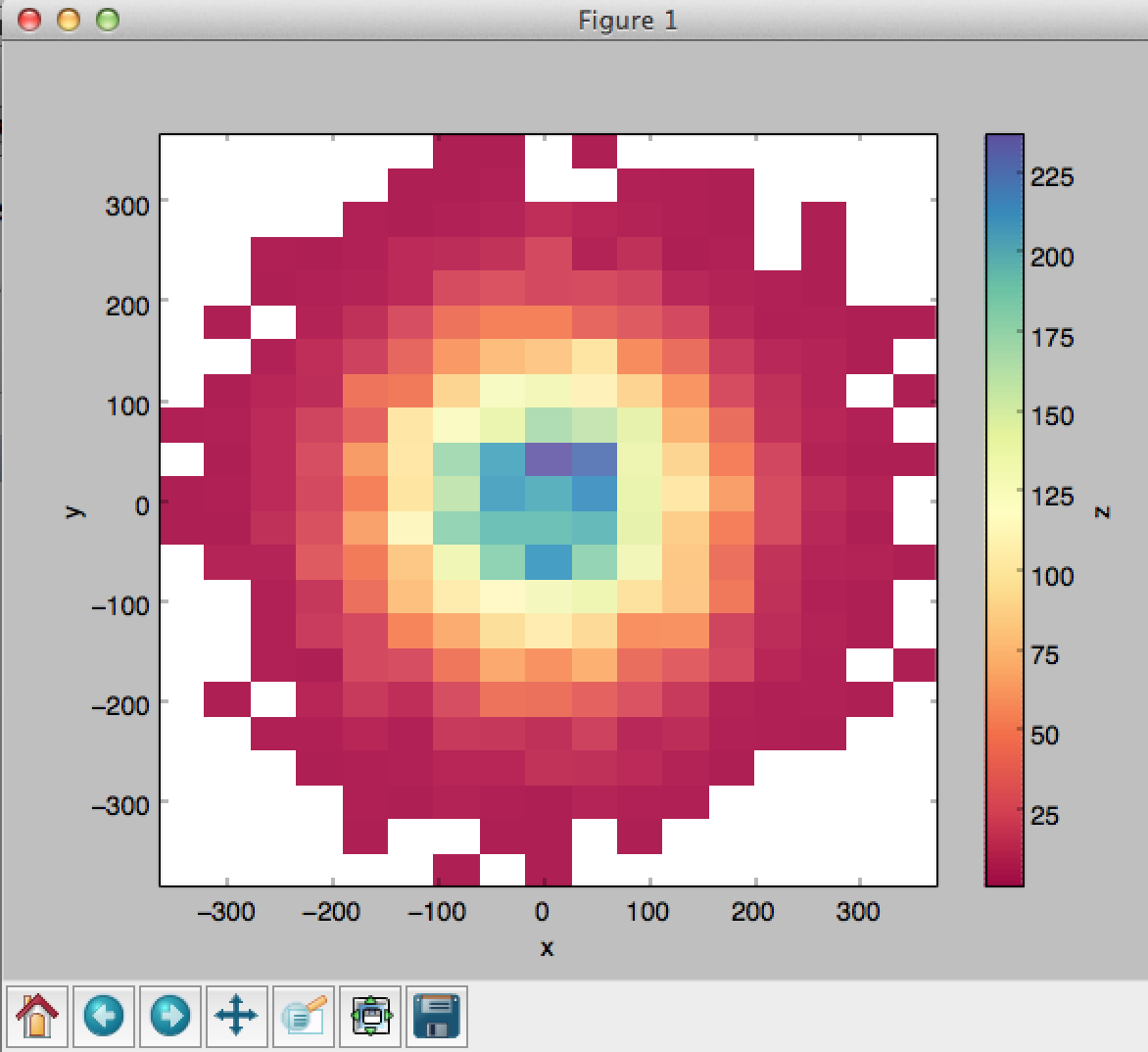(The current version of web application can NOT be used for computing 2-dimensional histogram.) See also 2-d kernel density estimation Q. I have used the Scott's method Optimal Bin Size= 3.49*s*n^(-1/3) , and obtained bin size that differs from the result obtained by the present method. A. They should be different. Three assumptions were made to obtain the Scott's result. First, the Scott's result is asymptotically true (i.e. it is true for large sample size n). Second, the scaling exponent -1/3 is true if the density is a smooth function. Third, the coefficient 3.49 was obtained, assuming the Gauss density function as a reference. The present method does not require these assumptions. Q.What is the assumption in your method? A. The method only assumes that the data are sampled independently each other (an assumption of a Poisson point process). No assumption was made for the underlying density function (for example, unimodality, continuity, existence of derivatives, etc.). Q. Is it different from the least squares cross-validation method? A. Yes. The above formula was derived under a Poisson point process assumption [see original paper], not by the cross-validation. As a result, the formulas and chosen bandwidths by the two methods are not identical. Q. What is specific about the Poisson assumption? A. In classical density estimation, the sampling size is fixed. Under the Poisson assumption, the total data size is not fixed, but assumed to obey a Poisson distribution. The MISE is an average error measured by the histograms with 'many' realizations of the experiment. Hence, the histograms constructed from a Poisson point events have more variability than the histograms constructed from samples with a fixed amount. This leads to a choice of wider optimal bin size for a histogram under a Poisson point process assumption. The difference becomes negligible as we increase the data size. Q. You provide the method for optimizing kernel density estimate, too. Which of the methods, a histogram or kernel, do you recommend for density estimation? A. We generally recommend to use the kernel density estimate. Please check the kernel density optimization page, too.   The FAQ includes my (HS) opinion. They are not opinions neither by my collaborators nor institutions I belong to.

## How it works

A bar-graph histogram is constructed simply by counting the number of events that belong to each bin of width. We divide the data range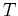intointervals with each length given by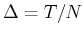. The number of events accumulated from all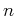sequences in the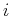th interval is counted as. The bar height at theth bin is given as.

In this thesis, we assess the goodness of the fit of the estimator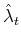to the underlying rate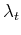over the data rangeby the mean integrated squared error (MISE),

 MISE(3.3)
whererefers to the expectation over different realization of point events, given.

By segmenting the rangeintointervals of size, the MISE defined in Eq.(3.3) can be rewritten as

 MISE(3.4)
whereis an empirical height of a bar-graph histogram atth bin. Hereafter we denote the average over those segmented rate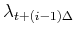as an average over an ensemble of (segmented) rate functions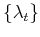defined in an interval of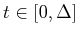:
 MISE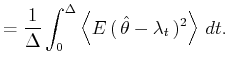(3.5)
The expectationnow refers to the average over the event count, or, given a rate function, or its mean value,. The MISE can be decomposed into two parts,
 MISE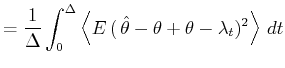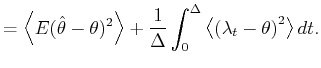(3.6)
The first and second terms are respectively the stochastic fluctuation of the estimator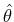around the expected mean rate, and the temporal fluctuation ofaround its meanover an interval of length, averaged over the segments.

The second term of Eq.(3.5) can further be decomposed into two parts,(3.7)
The first term in the rhs of Eq.( 3.7) represents a mean squared fluctuation of the underlying ratefrom the mean rate, and is independent of the bin size, because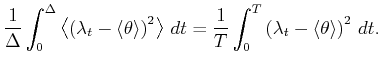(3.8)
We define a cost function by subtracting this term from the original MISE,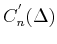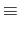MISE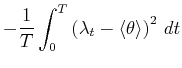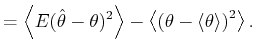(3.9)
The second term in Eq.( 3.9) represents the temporal fluctuation of the expected mean ratefor individual intervals of width. As the expected mean rate is not an observable quantity, we must replace the fluctuation of the expected mean rate with that of the observable estimator. Using the decomposition rule for an unbiased estimator (,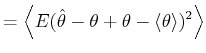(3.10)
the cost function is transformed into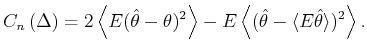(3.11)
Due to the assumed Poisson nature of the point process, the number of events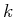counted in each bin obeys a Poisson distribution: the variance ofis equal to the mean. For the estimated rate defined as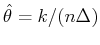, this variance-mean relation corresponds to(3.14)
By incorporating Eq.( 3.14) into Eq.(3.13), the cost function is given as a function of the estimator,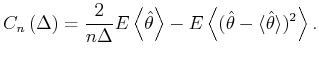(3.15)
The optimal bin size is obtained by minimizing the cost function:(3.17)

By replacingin Eq.(3.16) with the sample event counts, the method is converted into a user-friendly recipe summarized in the Method.

## Code

 YOUR CONTRIBUTION WELCOME! The codes include contribution by scientists from all over the world. If you could improve the code or translate the code to other languages, e.g., C/C++, please contact. It will be posted on this website with your name.

## A Sample Matlab Program

 The matlab function sshist.m is now available. [2008/11/19] To plot an optimized histogram of the data x (a vector contains samples as an element), type optN = sshist(x); hist(x,optN); Below is a simple sample program. %%%%%%%%%%%%%%%%%%%%%%%%%%%%%%%%%%%%%%%%%%%%%%%%%%%%%%%%%%%%%%%%%% % 2006 Author Hideaki Shimazaki % Department of Physics, Kyoto University % shimazaki at ton.scphys.kyoto-u.ac.jp % Please feel free to use/modify this program. % % Data: the duration for eruptions of % the Old Faithful geyser in Yellowstone National Park (in minutes) clear all; x = [4.37 3.87 4.00 4.03 3.50 4.08 2.25 4.70 1.73 4.93 1.73 4.62 ... 3.43 4.25 1.68 3.92 3.68 3.10 4.03 1.77 4.08 1.75 3.20 1.85 ... 4.62 1.97 4.50 3.92 4.35 2.33 3.83 1.88 4.60 1.80 4.73 1.77 ... 4.57 1.85 3.52 4.00 3.70 3.72 4.25 3.58 3.80 3.77 3.75 2.50 ... 4.50 4.10 3.70 3.80 3.43 4.00 2.27 4.40 4.05 4.25 3.33 2.00 ... 4.33 2.93 4.58 1.90 3.58 3.73 3.73 1.82 4.63 3.50 4.00 3.67 ... 1.67 4.60 1.67 4.00 1.80 4.42 1.90 4.63 2.93 3.50 1.97 4.28 ... 1.83 4.13 1.83 4.65 4.20 3.93 4.33 1.83 4.53 2.03 4.18 4.43 ... 4.07 4.13 3.95 4.10 2.27 4.58 1.90 4.50 1.95 4.83 4.12]; x_min = min(x); x_max = max(x); N_MIN = 4; % Minimum number of bins (integer) % N_MIN must be more than 1 (N_MIN > 1). N_MAX = 50; % Maximum number of bins (integer) N = N_MIN:N_MAX; % # of Bins D = (x_max - x_min) ./ N; % Bin Size Vector %%%%%%%%%%%%%%%%%%%%%%%%%%%%%%%%%%%%%%%%%%%%%%%%%%%%%%%%%%%%%%%%%% % Computation of the Cost Function for i = 1: length(N) edges = linspace(x_min,x_max,N(i)+1); % Bin edges ki = histc(x,edges); % Count # of events in bins ki = ki(1:end-1); k = mean(ki); % Mean of event count v = sum( (ki-k).^2 )/N(i); % Variance of event count C(i) = ( 2*k - v ) / D(i)^2; % The Cost Function end %%%%%%%%%%%%%%%%%%%%%%%%%%%%%%%%%%%%%%%%%%%%%%%%%%%%%%%%%%%%%%%%%% % Optimal Bin Size Selectioin [Cmin idx] = min(C); optD = D(idx); % *Optimal bin size edges = linspace(x_min,x_max,N(idx)+1); % Optimal segmentation %%%%%%%%%%%%%%%%%%%%%%%%%%%%%%%%%%%%%%%%%%%%%%%%%%%%%%%%%%%%%%%%%% % Display an Optimal Histogram and the Cost Function subplot(1,2,1); hist(x,edges); axis square; subplot(1,2,2); plot(D,C,'k.',optD,Cmin,'r*'); axis square; 

## A Sample R Program

 Copy and Paste a sample program sample.R, then run sshist(faithful[,1]) . Display code close  #%%%%%%%%%%%%%%%%%%%%%%%%%%%%%%%%%%%%%%%%%%%%%%%%%%%%%%%%%%%%%%%%% # 2006 Author Hideaki Shimazaki # Department of Physics, Kyoto University # shimazaki at ton.scphys.kyoto-u.ac.jp sshist <- function(x){ N <- 2: 100 C <- numeric(length(N)) D <- C for (i in 1:length(N)) { D[i] <- diff(range(x))/N[i] edges = seq(min(x),max(x),length=N[i]) hp <- hist(x, breaks = edges, plot=FALSE ) ki <- hp\$counts k <- mean(ki) v <- sum((ki-k)^2)/N[i] C[i] <- (2*k-v)/D[i]^2 #Cost Function } idx <- which.min(C) optD <- D[idx] edges <- seq(min(x),max(x),length=N[idx]) h = hist(x, breaks = edges ) rug(x) return(h) } 

## An Excel Program (contribution by Anil Kumar Tyagi)

 The following excel program that returns an optimal histogram is contributed by Anil Kumar Tyagi (ITER-India). sshist_tyagi.xlsm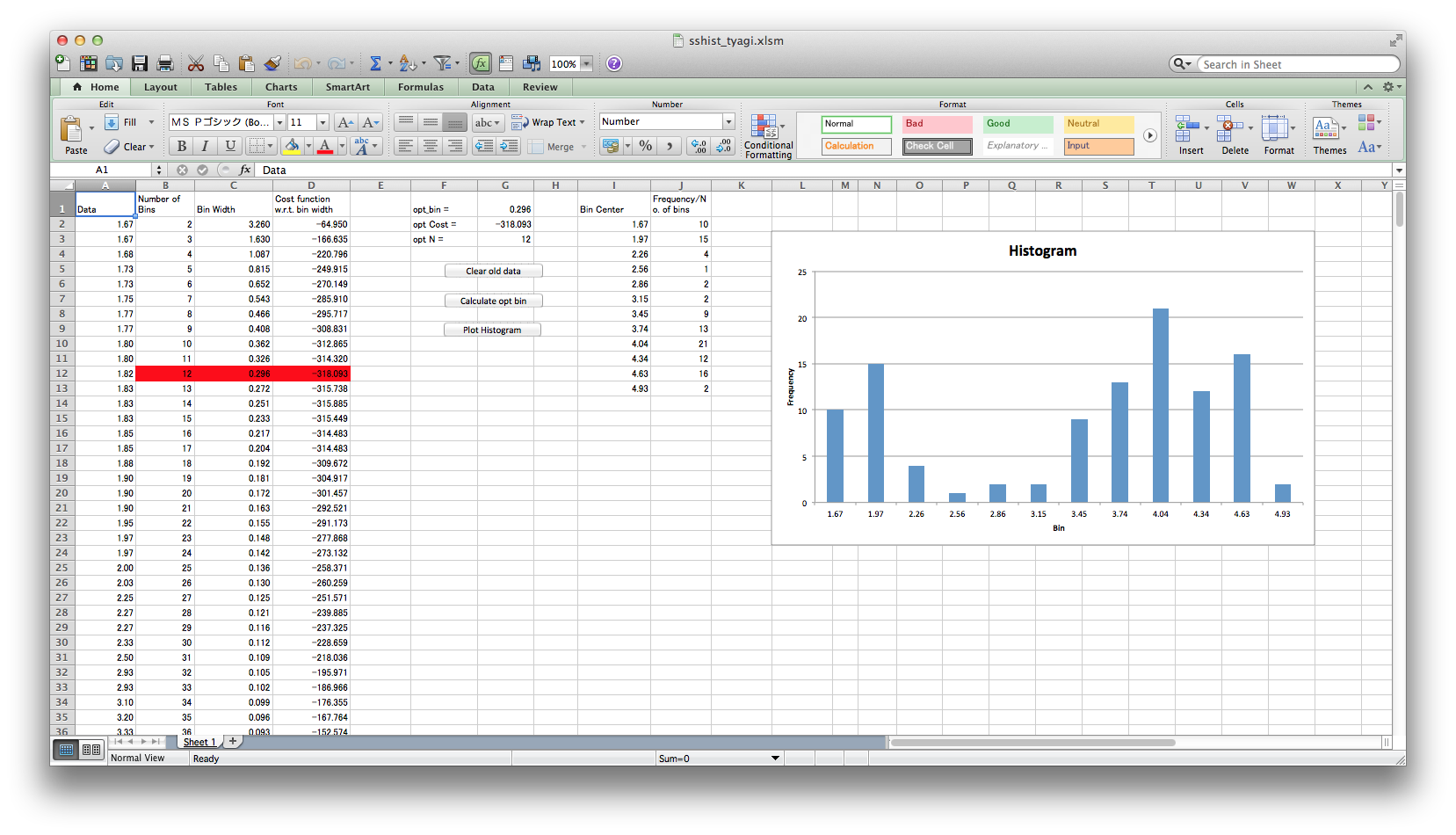## (contribution by Érbet Almeida Costa and Takuma Torii )

 A sample Python program contributed by Érbet Almeida Costa. histsample.py More sample Python programs contributed by Takuma Torii. (Feb. 24, 2013) histsample_purepython.py (code without numpy) """ Created on Thu Oct 25 11:32:47 2012 Histogram Binwidth Optimization Method Shimazaki and Shinomoto, Neural Comput 19 1503-1527, 2007 2006 Author Hideaki Shimazaki, Matlab Department of Physics, Kyoto University shimazaki at ton.scphys.kyoto-u.ac.jp Please feel free to use/modify this program. Version in python adapted Érbet Almeida Costa Data: the duration for eruptions of the Old Faithful geyser in Yellowstone National Park (in minutes) or normal distribuition. Version in python adapted Érbet Almeida Costa Bugfix by Takuma Torii 2.24.2013 """ import numpy as np from numpy import mean, size, zeros, where, transpose from numpy.random import normal from matplotlib.pyplot import hist from scipy import linspace import array from matplotlib.pyplot import figure, plot, xlabel, ylabel,\ title, show, savefig, hist x = normal(0, 100, 1e2) # Generate n pseudo-random numbers whit(mu,sigma,n) #x = [4.37,3.87,4.00,4.03,3.50,4.08,2.25,4.70,1.73,4.93,1.73,4.62,\ #3.43,4.25,1.68,3.92,3.68,3.10,4.03,1.77,4.08,1.75,3.20,1.85,\ #4.62,1.97,4.50,3.92,4.35,2.33,3.83,1.88,4.60,1.80,4.73,1.77,\ #4.57,1.85,3.52,4.00,3.70,3.72,4.25,3.58,3.80,3.77,3.75,2.50,\ #4.50,4.10,3.70,3.80,3.43,4.00,2.27,4.40,4.05,4.25,3.33,2.00,\ #4.33,2.93,4.58,1.90,3.58,3.73,3.73,1.82,4.63,3.50,4.00,3.67,\ #1.67,4.60,1.67,4.00,1.80,4.42,1.90,4.63,2.93,3.50,1.97,4.28,\ #1.83,4.13,1.83,4.65,4.20,3.93,4.33,1.83,4.53,2.03,4.18,4.43,\ #4.07,4.13,3.95,4.10,2.27,4.58,1.90,4.50,1.95,4.83,4.12] x_max = max(x) x_min = min(x) N_MIN = 4 #Minimum number of bins (integer) #N_MIN must be more than 1 (N_MIN > 1). N_MAX = 50 #Maximum number of bins (integer) N = range(N_MIN,N_MAX) # #of Bins N = np.array(N) D = (x_max-x_min)/N #Bin size vector C = zeros(shape=(size(D),1)) #Computation of the cost function for i in xrange(size(N)): edges = linspace(x_min,x_max,N[i]+1) # Bin edges ki = hist(x,edges) # Count # of events in bins ki = ki k = mean(ki) #Mean of event count v = sum((ki-k)**2)/N[i] #Variance of event count C[i] = (2*k-v)/((D[i])**2) #The cost Function #Optimal Bin Size Selection cmin = min(C) idx = where(C==cmin) idx = int(idx) optD = D[idx] edges = linspace(x_min,x_max,N[idx]+1) fig = figure() ax = fig.add_subplot(111) ax.hist(x,edges) title(u"Histogram") ylabel(u"Frequency") xlabel(u"Value") savefig('Hist.png') fig = figure() plot(D,C,'.b',optD,cmin,'*r') savefig('Fobj.png') 

## (contribution by Cristóvão Freitas Iglesias Junior)

 A sample Python program for 2D histogram contributed by Cristóvão Freitas Iglesias Junior. (Oct. 19, 2013) histsample2d_iglesias.py

## A Sample Mathematica Program

 Copy and Paste a sample program sample.nb, then ctrl+shift. Display code close \!$$<< \ GraphicsGraphics\n << StatisticsDescriptiveStatistics\[IndentingNewLine] \(x = {4.37, 3.87, 4.00\ , 4.03, \ 3.50, \ 4.08, \ 2.25, \ 4.70, \ 1.73, \ 4.93, \ 1.73, \ 4.62, \ 3.43, \ 4.25, \ 1.68, \ 3.92, \ 3.68, \ 3.10, \ 4.03, \ 1.77, \ 4.08, \ 1.75, \ 3.20, \ 1.85, 4.62, \ 1.97, \ 4.50, \ 3.92, \ 4.35, \ 2.33, \ 3.83\ , 1.88, \ 4.60, \ 1.80, \ 4.73, \ 1.77, \ 4.57, \ 1.85, \ 3.52, \ 4.00, \ 3.70, \ 3.72, \ 4.25, \ 3.58, \ 3.80, \ 3.77, \ 3.75, \ 2.50, 4.50, \ 4.10, \ 3.70, \ 3.80, \ 3.43, \ 4.00, \ 2.27, \ 4.40, \ 4.05, \ 4.25, \ 3.33, \ 2.00, \ 4.33, \ 2.93, \ 4.58, \ 1.90, \ 3.58, \ 3.73, \ 3.73, \ 1.82, \ 4.63, \ 3.50, \ 4.00, \ 3.67, \ 1.67, \ 4.60, \ 1.67, \ 4.00, \ 1.80, \ 4.42, \ 1.90\ , 4.63, \ 2.93, \ 3.50, \ 1.97, \ 4.28, \ 1.83, \ 4.13, \ 1.83, \ 4.65, \ 4.20, \ 3.93, \ 4.33, \ 1.83, \ 4.53, \ 2.03, \ 4.18, \ 4.43, \ 4.07, \ 4.13, \ 3.95, \ 4.10, \ 2.27, \ 4.58, \ 1.90, \ 4.50, \ 1.95, \ 4.83, \ 4.12};$$\[IndentingNewLine] c = {}; Δ = {}; \ d = {}; p = {};\[IndentingNewLine] For[i = 1, i < 50, $$i++$$, \[IndentingNewLine]n = i + 3; \[IndentingNewLine]d = Append[d, $$(Max[x] - Min[x])$$/n]; \[IndentingNewLine]ki = BinCounts[ x, {Min[x], Max[x], d[$$[i]$$]}]; \[IndentingNewLine] (*Cost\ Function\*) \[IndentingNewLine]k\ = \ Mean[ki]; \[IndentingNewLine]v\ = \ VarianceMLE[ki]; \[IndentingNewLine]c = Append[c, $$(2 k - v)$$/d[$$[i]$$]\^2]; \[IndentingNewLine]p = Append[p, {d[$$[i]$$], c[$$[i]$$]}];\[IndentingNewLine]]\[IndentingNewLine] (*Optimal\ Bin\\ Size\ Selection*) \[IndentingNewLine] $$idx = \(Ordering[c]$$[$$$$];\)\[IndentingNewLine] $$d\^*$$ = d[$$[idx]$$]\[IndentingNewLine] $$edges = {};$$\[IndentingNewLine] $$edges = Do[Append[edges, Min[x] + \(d\^*$$*i], {i, 0, idx + 3}];\)\[IndentingNewLine] (*Display\ an\ Optimized\ Histogram\*) \[IndentingNewLine] $$Histogram[x, \ HistogramRange -> {Min[x], Max[x]}, HistogramCategories -> edges];$$\[IndentingNewLine] $$ListPlot[p];$$\)

## IDL Functions (contribution by Dr. Shigenobu Hirose)

 The following IDL programs were contributed by Dr. Shigenobu Hirose at JAMSTEC. sshist.pro 1-dimensional histogram optimization. Display code close; Author: Shigenobu Hirose at JAMSTEC ; based on original paper ; Shimazaki and Shinomoto, Neural Computation 19, 1503-1527, 2007 ; function sshist, data, x=x, cost=cost, nbin=nbin COMPILE_OPT idl2 nbin_min = 2 nbin_max = 200 ntrial = nbin_max - nbin_min + 1 nbin = INDGEN(ntrial) + nbin_min delta = FLTARR(ntrial) cost = FLTARR(ntrial) for n = 0, ntrial-1 do begin delta[n] = (MAX(data) - MIN(data)) / (nbin[n] - 1) k = HISTOGRAM(data, nbins=nbin[n]) kmean = MEAN(k) kvari = MEAN((k - kmean)^2) cost[n] = (2. * kmean - kvari) / delta[n]^2 endfor n = (WHERE(cost eq MIN(cost))) k = HISTOGRAM(data, nbins=nbin[n], locations=x) return, k end    sshist_2d.pro 2-dimensional histogram optimization. Display code close; Author: Shigenobu Hirose at JAMSTEC ; based on original paper ; Shimazaki and Shinomoto, Neural Computation 19, 1503-1527, 2007 ; function sshist_2d, data1, data2, x1=x1, x2=x2, cost=cost, nbin=nbin COMPILE_OPT idl2 nbin_min = 2 nbin_max = 200 ntrial = nbin_max - nbin_min + 1 nbin = INDGEN(ntrial) + nbin_min delta1 = FLTARR(ntrial) delta2 = FLTARR(ntrial) cost = FLTARR(ntrial) for n = 0, ntrial-1 do begin delta1[n] = (MAX(data1) - MIN(data1)) / (nbin[n] - 1) * (1. - (MACHAR()).EPS) delta2[n] = (MAX(data2) - MIN(data2)) / (nbin[n] - 1) * (1. - (MACHAR()).EPS) k = HIST_2D(data1, data2, bin1=delta1[n], bin2=delta2[n], min1=MIN(data1), min2=MIN(data2)) kmean = MEAN(k) kvari = MEAN((k - kmean)^2) cost[n] = (2. * kmean - kvari) / (delta1[n] * delta2[n])^2 endfor n = (WHERE(cost eq MIN(cost))) k = HIST_2D(data1, data2, bin1=delta1[n], bin2=delta2[n], min1=MIN(data1), min2=MIN(data2)) x1 = FINDGEN(nbin[n]) * delta1[n] + MIN(data1) x2 = FINDGEN(nbin[n]) * delta2[n] + MIN(data2) return, k end `

## Java program

 The web appliation is written in Java. Here are the core codes, BinSelection.java and DrawHistogram.java . (BarHisto.java) They work with Javascript on this page.

## Links

© 2006-11 Hideaki Shimazaki All rights reserved.